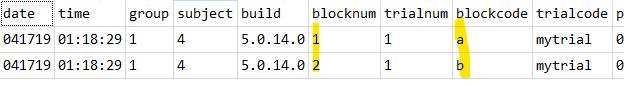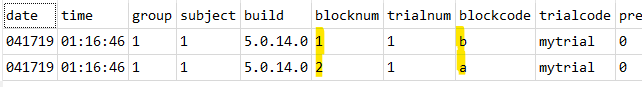## Reading order of blocks in data

 Author Message linatetAssociate MemberGroup: Awaiting Activation Posts: 3, Visits: 20 Hi,My version is actually 5, but for some reason I am not allowed to post on the Inquisit 5 forum.I want to know how I can identify in the data the order participants saw the blocks. This is the part of the script I divide conditions:/ subjects = (1 of 8)/ groupassignment = random/ blocks = [1=inst; 2=intro_implicit; 3=test; 4=category_explicit; 5=demographics]/ subjects = (2 of 8)/ groupassignment = random/ blocks = [1=inst; 2=intro_implicit; 3=test; 4=faces_explicit; 5=demographics]/ subjects = (3 of 8)/ groupassignment = random/ blocks = [1=inst; 2=intro_implicit; 3=category_implicit; 4=category_explicit; 5=demographics]/ subjects = (4 of 8)/ groupassignment = random/ blocks = [1=inst; 2=intro_implicit; 3=category_implicit; 4=faces_explicit; 5=demographics]/ subjects = (5 of 8)/ groupassignment = random/ blocks = [1=inst; 2=category_explicit; 3=intro_implicit; 4=test; 5=demographics]/ subjects = (6 of 8)/ groupassignment = random/ blocks = [1=inst; 2=faces_explicit; 3=intro_implicit; 4=test; 5=demographics]/ subjects = (7 of 8)/ groupassignment = random/ blocks = [1=inst; 2=category_explicit; 3=intro_implicit; 4=category_implicit; 5=demographics]/ subjects = (8 of 8)/ groupassignment = random/ blocks = [1=inst; 2=faces_explicit; 3=intro_implicit; 4=category_implicit; 5=demographics]Note that the assignment is random and not by subject number or condition. How can I see in the study data the order participants saw the blocks? Thank you so much!! Edited 8 Months Ago by linatet DaveSupreme BeingGroup: Administrators Posts: 9.9K, Visits: 49K +xlinatet - Tuesday, April 16, 2019Hi,My version is actually 5, but for some reason I am not allowed to post on the Inquisit 5 forum.I want to know how I can identify in the data the order participants saw the blocks. This is the part of the script I divide conditions:/ subjects = (1 of 8)/ groupassignment = random/ blocks = [1=inst; 2=intro_implicit; 3=test; 4=category_explicit; 5=demographics]/ subjects = (2 of 8)/ groupassignment = random/ blocks = [1=inst; 2=intro_implicit; 3=test; 4=faces_explicit; 5=demographics]/ subjects = (3 of 8)/ groupassignment = random/ blocks = [1=inst; 2=intro_implicit; 3=category_implicit; 4=category_explicit; 5=demographics]/ subjects = (4 of 8)/ groupassignment = random/ blocks = [1=inst; 2=intro_implicit; 3=category_implicit; 4=faces_explicit; 5=demographics]/ subjects = (5 of 8)/ groupassignment = random/ blocks = [1=inst; 2=category_explicit; 3=intro_implicit; 4=test; 5=demographics]/ subjects = (6 of 8)/ groupassignment = random/ blocks = [1=inst; 2=faces_explicit; 3=intro_implicit; 4=test; 5=demographics]/ subjects = (7 of 8)/ groupassignment = random/ blocks = [1=inst; 2=category_explicit; 3=intro_implicit; 4=category_implicit; 5=demographics]/ subjects = (8 of 8)/ groupassignment = random/ blocks = [1=inst; 2=faces_explicit; 3=intro_implicit; 4=category_implicit; 5=demographics]Note that the assignment is random and not by subject number or condition. How can I see in the study data the order participants saw the blocks? Thank you so much!!You can do one of four things:(1) Look at the raw data files, where you'll directly see in which order the blocks were run.(2) Choose a different /groupassignment method (subjectnumber or groupnumber), then you can directly infer the order from the automatically logged subject or group variable in your data files (see https://www.millisecond.com/forums/Topic13856.aspx).(3) Log script.groupassignmentcode to the data file(s) as in/ subjects = (1 of 2)/ groupassignment = random/ blocks = [1=a; 2=b]/ subjects = (2 of 2)/ groupassignment = random/ blocks = [1=b; 2=a]/ trials = [1=mytrial]/ trials = [1=mytrial]/ trialduration = 10/ columns = (script.groupassignmentcode)and then you can apply the same method detailed under (2) to the groupassignmentcode variable (https://www.millisecond.com/support/docs/v5/html/language/properties/groupassignmentcode.htm).(4) Record the order in a entry /onexptbegin and log that value to the data file:/ blockorder = 0/ onexptbegin = [values.blockorder = 1;]/ subjects = (1 of 2)/ groupassignment = random/ blocks = [1=a; 2=b]/ onexptbegin = [values.blockorder = 2;]/ subjects = (2 of 2)/ groupassignment = random/ blocks = [1=b; 2=a]/ trials = [1=mytrial]/ trials = [1=mytrial]/ trialduration = 10/ columns = (values.blockorder) linatetAssociate MemberGroup: Awaiting Activation Posts: 3, Visits: 20 Thank you for the reply!Regarding number 1, how do I see the block order in the data file? Sorry if this is a simple question, but I can't find it. I can tell which blocks participants saw based on the answers, but not which order. DaveSupreme BeingGroup: Administrators Posts: 9.9K, Visits: 49K +xlinatet - Tuesday, April 16, 2019Thank you for the reply!Regarding number 1, how do I see the block order in the data file? Sorry if this is a simple question, but I can't find it. I can tell which blocks participants saw based on the answers, but not which order.Typically, your *raw* data files will have "blockcode" and "blocknum" columns, which you can read top to down:Here the order is A -> BAnd here the order is B -> AEdited 8 Months Ago by Dave linatetAssociate MemberGroup: Awaiting Activation Posts: 3, Visits: 20 Thank you so much!! You are a great help.